﻿ 基于视觉/力觉的机器人协同缝制系统
 机器人2022, Vol. 44Issue (3): 352-3600

FU Tianyu, LI Fengming, CUI Tao, SONG Rui, WANG Yanhong. A Robotic Collaborative Sewing System Based on Visual and Force Perception[J]. ROBOT, 2022, 44(3): 352-360.1. 山东大学控制科学与工程学院，山东 济南 250061;
2. 山东建筑大学信息与电气工程学院，山东 济南 250101

A Robotic Collaborative Sewing System Based on Visual and Force Perception
FU Tianyu1 , LI Fengming1,2 , CUI Tao1 , SONG Rui1, WANG Yanhong1
1. School of Control Science and Engineering, Shandong University, Ji'nan 250061, China;
2. School of Information and Electrical Engineering, Shandong Jianzhu University, Ji'nan 250101, China
Abstract: The automation of the sewing process in garment manufacturing industry is at a low level. For this problem, an intelligent sewing system in which the robot cooperates with the sewing machine based on visual and force perception information is designed and built to realize automatic sewing of various polygon fabrics. A fabric feature extraction method based on corner detection is used to realize trajectory planning for robotic sewing. Based on the hybrid position/force control strategy, collaborative operation of the robot and the sewing machine in sewing process is realized to ensure the sewing quality. Finally, the effect of the single-side robotic sewing and the overall effect of sewing the polygon fabric edge at different speeds are tested. The experimental results show that the designed robotic sewing system can realize the collaborative sewing of the two machines at different speeds. The error of the distance from the stitch to the fabric edge is less than 2 mm, the error of stitch distance is less than 0.5 mm, and the error of the total stitch length is less than 1.6 cm.
Keywords: robotic sewing    trajectory planning    hybrid position/force control    collaborative control

1 引言（Introduction）

2 机器人协同缝制系统设计（Design of the robotic collaborative sewing system）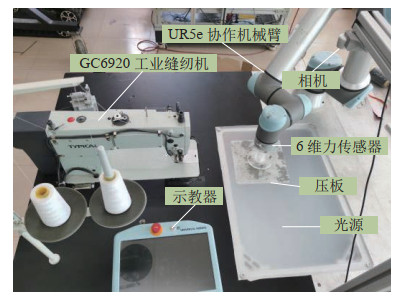图 1 机器人缝制整体实验平台 Fig.1 The overall experimental platform for robotic sewing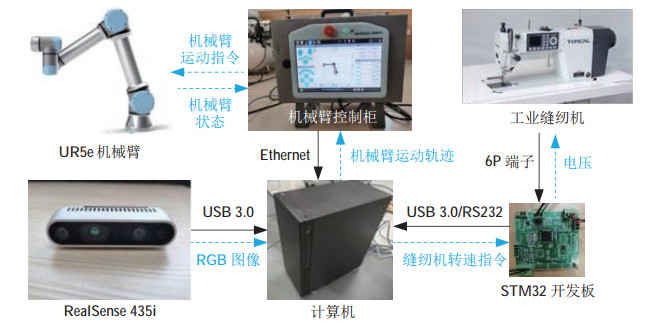图 2 机器人缝制平台数据流 Fig.2 The data stream of robotic sewing platform
2.1 缝制线迹规划模块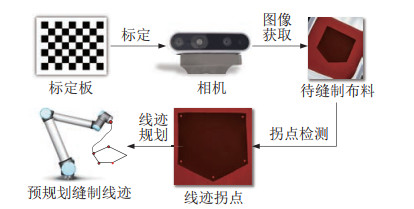图 3 缝制线迹规划模块 Fig.3 The module for sewing stitch planning
2.2 协同控制缝制模块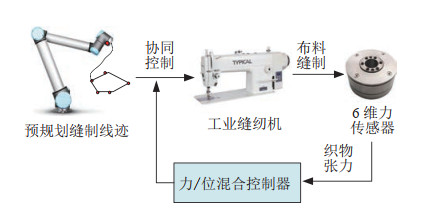图 4 机器人协同控制缝制模块 Fig.4 Collaborative control module for robotic sewing
3 基于拐点检测的机器人缝制线迹规划（Planning of the robotic sewing stitch based on corner detection）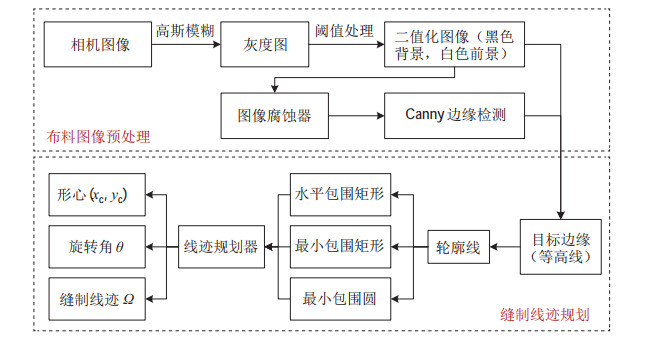图 5 基于拐点检测的机器人缝制线迹规划流程 Fig.5 Planning of the robotic sewing stitch based on corner detection
3.1 待缝织物图像预处理

 \begin{align} g(x, y)=\sum _{u=-r}^r \sum _{v=-r}^r s(x+u, y+v)f(u, v) \end{align} (1)

 \begin{align} A-B=\{x|B_{x} \subseteq A\} \end{align} (2)

3.2 缝制线迹规划

 \begin{align} \varOmega =\{P, S\} \end{align} (3)

 \begin{align} s_{i-1}^{i}:\; \; A_{i} x+B_{i} y+C_{i} =0 \end{align} (4)

 \begin{align} s_{i-1}^{i}:\; \; \frac{y-y_{i-1}} {y_{i} -y_{i-1}} =\frac{x-x_{i-1}} {x_{i} -x_{i-1}} \end{align} (5)

 \begin{align} s_{n}^{0}: \; \; \frac{y-y_{n}} {y_{0} -y_{n}} =\frac{x-x_{n}} {x_{0} -x_{n}} \end{align} (6)

 $\begin{equation} \theta_{i} = \begin{cases} 90^{\circ} -\arctan k_{i} , & k_{i-1} =\infty \\ \arctan k_{i-1} -90^{\circ}, & k_{i} =\infty \\ \arctan \dfrac{k_{i} -k_{i-1}} {1+k_{i-1} \cdot k_{i}}, & k_{i-1} \cdot k_{i} \ne -1 \\ 90^{\circ}, & k_{i-1} \cdot k_{i} =-1 \end{cases} \\ \end{equation}$ (7)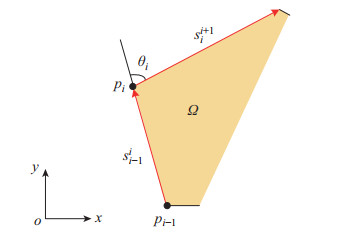图 6 缝制路径与缝制拐点示意图 Fig.6 The schematic of the sewing trajectory and the corner
4 基于力/位混合控制的机器人协同缝制（The robotic collaborative sewing based on hybrid position/force control） 4.1 缝制过程任务分析

 \begin{align} \begin{cases} v_{{\rm c}x} =0 \\ v_{{\rm c}z} =0 \\ f_{{\rm c}x} =0 \\ f_{{\rm c}y} =0 \end{cases} \end{align} (8)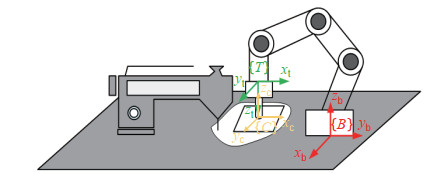图 7 缝制任务空间示意图 Fig.7 Schematic of the sewing task space

 \begin{align} \begin{cases} v_{{\rm c}y} =v \\ f_{{\rm c}z} =f \end{cases} \end{align} (9)

4.2 力/位混合控制 4.2.1 位置控制

 \begin{align} \begin{cases} {}^{T}\mathit{\boldsymbol{x}}_{\rm d} ={}_{C}^{T} \mathit{\boldsymbol{P}}{}^{C}\mathit{\boldsymbol{x}}_{\rm d} \\ {}^{T}\dot{\mathit{\boldsymbol{x}}}_{\rm d} =\mathit{\boldsymbol{J}}_{1} (\theta){}^{C}\dot{\mathit{\boldsymbol{x}}}_{\rm d} \\ {}^{T}\ddot{\mathit{\boldsymbol{x}}}_{\rm d} =\dot{\mathit{\boldsymbol{J}}}_{1} (\theta){}^{C}\dot{\mathit{\boldsymbol{x}}}_{\rm d} +\mathit{\boldsymbol{J}}_{1} (\theta){}^{C}\ddot{\mathit{\boldsymbol{x}}}_{\rm d} \end{cases} \end{align} (10)

 \begin{align} \mathit{\boldsymbol{u}}_{\rm p} =K_{\rm Pp} ({}^{T}\mathit{\boldsymbol{x}}_{\rm d} -{}^{T}\mathit{\boldsymbol{x}})^{\rm T}+K_{\rm Pd} ({}^{T}\dot{\mathit{\boldsymbol{x}}}_{\rm d} -{}^{T}\dot{\mathit{\boldsymbol{x}}})+\ddot{\mathit{\boldsymbol{x}}}_{\rm d} \end{align} (11)

 \begin{align} \ddot{\theta} =\mathit{\boldsymbol{J}}_{0}^{-1} (\theta)({}^{T}\ddot{\mathit{\boldsymbol{x}}}-\dot{\mathit{\boldsymbol{J}}}_{0} (\theta)\dot{\theta} ) \end{align} (13)

 \begin{align} \mathit{\boldsymbol{\tau}} =\mathit{\boldsymbol{M}}(\theta)\mathit{\boldsymbol{J}}_{0}^{-1} (\theta)({\mathit{\boldsymbol{u}}-\dot{\mathit{\boldsymbol{J}}}_{0} (\theta)\dot{\theta}})+\mathit{\boldsymbol{V}}(\theta, \dot{\theta})+\mathit{\boldsymbol{G}}(\theta) \end{align} (14)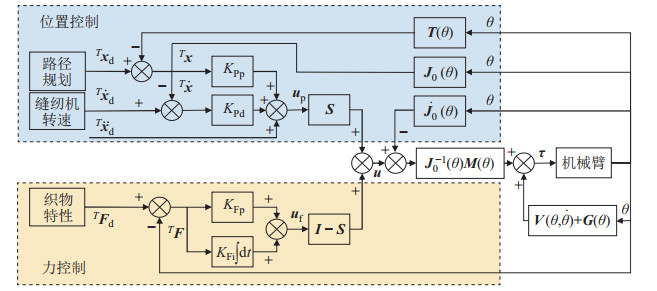图 8 基于力/位混合控制的机器人协同缝制控制框图 Fig.8 The schematic of robotic collaborative sewing based hybrid position/force control
5 实验验证与讨论（Experimental validation and discussion）

5.1 缝制轨迹规划 5.1.1 相机标定

 \begin{align*} \mathit{\boldsymbol{f}} & =\begin{bmatrix} 1372.3 & 0 & 954.1 \\ 0 & 1374.1 & 547.9 \\ 0 & 0 & 1 \end{bmatrix} \\ \mathit{\boldsymbol{K}} & =\begin{bmatrix} 0.9997 & 0.0027 & 0.0242 & -258.9438 \\ 0.0027 & {-1} & -0.0002 & -430.1141 \\ 0.0242 & 0.0003 & -0.9997 & 578.5774 \\ 0 & 0 & 0 & 1 \end{bmatrix} \end{align*}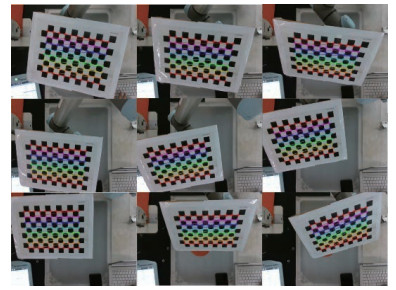图 9 标定过程 Fig.9 The calibration process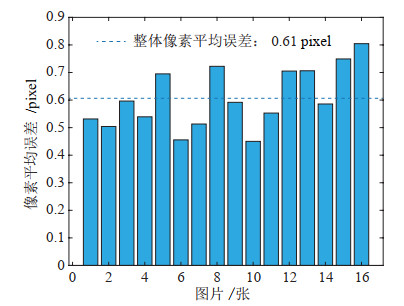图 10 标定实验结果 Fig.10 Results of the calibration experiment
5.1.2 轨迹规划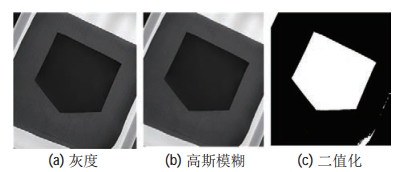图 11 织物图像预处理 Fig.11 Preprocessing of the fabric image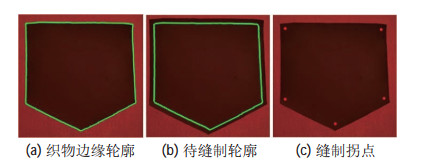图 12 织物缝制轨迹特征提取 Fig.12 The feature extraction of fabric sewing trajectory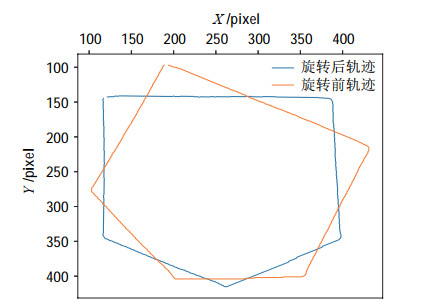图 13 机器人缝制轨迹 Fig.13 The trajectory of robotic sewing
5.2 协同控制缝制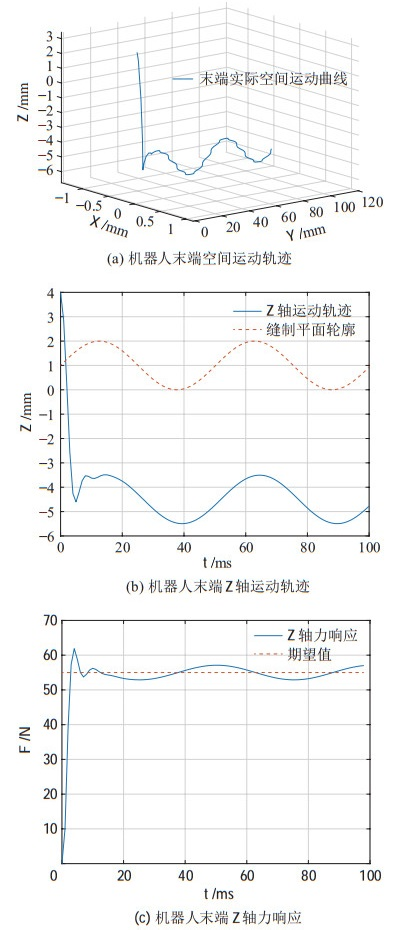图 14 协同控制仿真实验结果 Fig.14 The simulation results of collaborative control
5.3 机器人缝制系统结果 5.3.1 单边缝制实验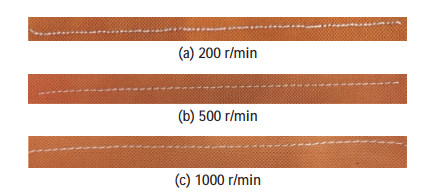图 15 单边缝纫结果 Fig.15 The results of single-side sewing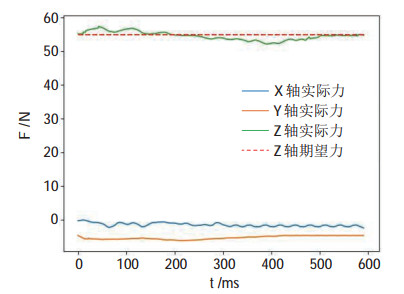图 16 机器人缝制过程中的末端力 Fig.16 The end force in robotic sewing process表 1 单边缝制数据 Tab. 1 The data of single-side sewing
5.3.2 多边缝制实验图 17 不规则多边形缝制效果 Fig.17 The sewing result of irregular polygons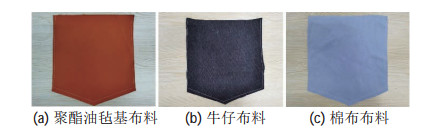图 18 不同性质织物缝制效果 Fig.18 The sewing result of different fabrics
6 结论（Conclusion）

  张秀芳, 路晨, 唐茂勇. 我国服装行业的现状及发展趋势[J]. 中国纤检, 2020(Z1): 160-163. Zhang X F, Lu C, Tang M Y. The present situation and development trend of China's garment industry[J]. China Fiber Inspection, 2020(Z1): 160-163.  Nayak R, Padhye R. Introduction to automation in garment manufacturing[M]//Automation in Garment Manufacturing. Sawston, UK: Woodhead Publishing, 2018: 1-27.  周晓钰, 朱颖, 孙银银. 服装智能制造的发展趋势[J]. 轻纺工业与技术, 2021, 50(8): 65-67. Zhou X Y, Zhu Y, Sun Y Y. The development trend of intelligent garment manufacturing[J]. Light and Textile Industry and Technology, 2021, 50(8): 65-67. DOI:10.3969/j.issn.2095-0101.2021.08.029  Matheson E, Minto R, Zampieri E G G, et al. Human-robot collaboration in manufacturing applications: A review[J]. Robotics, 2019, 8(4): 100. DOI:10.3390/robotics8040100  墨影, 孟庆杰. 服装智能制造进行时[J]. 纺织机械, 2016(6): 28-29. Mo Y, Meng Q J. Garment intelligent manufacturing is ongoing[J]. Textile Machinery, 2016(6): 28-29.  Gershon D, Porat I. Vision servo control of a robotic sewing system[C]//IEEE International Conference on Robotics and Automation. Piscataway, USA: IEEE, 1988: 1830-1835.  Paraskevi Z. Robot handling fabrics towards sewing using computational intelligence methods[M]//Robotic Systems. London, UK: IntechOpen, 2012: 61-84.  Schrimpf J. Automated sewing using conveyor belts[C]//IEEE 21st International Conference on Emerging Technologies and Factory Automation. Piscataway, USA: IEEE, 2016. DOI: 10.1109/ETFA.2016.7733653.  闻力生. 人工智能在服装智能制造中的应用[J]. 纺织高校基础科学学报, 2020, 33(2): 30-36. Wen L S. Application of artificial intelligence in garment intelligent manufacturing[J]. Basic Sciences Journal of Textile Universities, 2020, 33(2): 30-36.  高盼. Delta机器人扣眼缝制技术研究[D]. 西安: 西安工程大学, 2019. Gao P. Research on seaming technology of Delta robot[D]. Xi'an: Xi'an Polytechnic University, 2019.  Parker J K, Dubey R, Paul F W, et al. Robotic fabric handling for automating garment manufacturing[J]. Journal of Engineering for Industry, 1983, 105(1): 21-26.  Hou Y C, Sahari K S M, How D N T. A review on modeling of flexible deformable object for dexterous robotic manipulation[J]. International Journal of Advanced Robotic Systems, 2019, 16(3). DOI:10.1177/1729881419848894  Sanchez J, Corrales J-A, Bouzgarrou B-C, et al. Robotic manipulation and sensing of deformable objects in domestic and industrial applications: A survey[J]. International Journal of Robotics Research, 2018, 37(7): 688-716.  Jia B, Hu Z, Pan J, et al. Manipulating highly deformable materials using a visual feedback dictionary[C]//IEEE International Conference on Robotics and Automation. Piscataway, USA: IEEE, 2018: 239-246.  Sun L, Aragon-Camarasa G, Rogers S, et al. Autonomous clothes manipulation using a hierarchical vision architecture[J]. IEEE Access, 2018, 6: 76646-76662.  Fung E H K, Yuen C W M, Hau L C, et al, A robot system for the control of fabric tension for inspection[C]//ASME International Mechanical Engineering Congress and Exposition. New York, USA: ASME, 2007: 813-819.  Kruse D, Radke R J, Wen J T. Collaborative human-robot manipulation of highly deformable materials[C]//IEEE International Conference on Robotics and Automation. Piscataway, USA: IEEE, 2015: 3782-3787.  邓超, 刘岩岩. 基于边缘检测的斜纹布匹瑕疵检测[J]. 测控技术, 2018, 37(12): 110-113. Den C, Liu Y Y. Twill fabric detection based on edge detection[J]. Measurement and Control Technology, 2018, 37(12): 110-113.  Guan M, Zhong Z Z, Rui Y N. Automatic defect segmentation for plain woven fabric images[C]//International Conference on Communications, Information System and Computer Engineering. Piscataway, USA: IEEE, 2019: 465-468.  Ma R J, Deng S P, Sun H C, et al. An algorithm for fabric defect detection based on adaptive Canny operator[C]//International Conference on Intelligent Computing, Automation and Systems. Piscataway, USA: IEEE, 2019: 475-481.  吴柳波, 李新荣, 杜金丽. 基于轮廓提取的缝纫机器人运动轨迹规划研究进展[J]. 纺织学报, 2021, 42(4): 191-200. Wu L B, Li X R, Du J L. Research progress of motion trajectory planning of sewing robot based on contour extraction[J]. Journal of Textile Research, 2021, 42(4): 191-200.  刘锋. 图解服装裁剪与缝纫工艺: 基础篇[M]. 北京: 化学工业出版社, 2020. Liu F. Graphic illustration of clothing cutting and sewing technology: The basics[M]. Beijing: Chemical Industry Press, 2020.